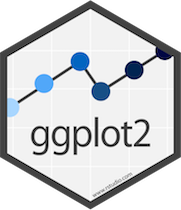Working with ggplots in R

ggplot or grammar of graphics plots, is built for making profressional looking, plots quickly with minimal code.General

• Cheatsheet - basic layers
• ggplot basics and function reference

• geom layers

• stat layers

• position adjustment layers

• annotations layers

• aesthetics layers

• scale layers

• axes and legends

• facetting and facetting layers

• coordinate systems

• themes

• programming with ggplot2

• vector helpers

• built in datasets

• autoplot and fortify

Colors

• quick reference for colors in ggplot2

• predefined colour names

• various graphs you can make with ggplot2 with options for customization

• bar, line, distributions, scatterplots

• titles, axes, legends, lines, facets, multiple graphs on one page, colors

Legends

• cheatsheet for customizing graphics and legends for ggplot2
• legend basics for ggplot2
• legend customization in ggplot2

Themes

• modifying components of a theme in ggplot2
• Using a variable value in a title for ggplot2

Multivariate Analysis

• using lattice for multivariate data visualization with ggplot2
• arguments and aesthetics for geomdensity() and statdensity()
• histograms in ggplot2 with customization options
• plotting means and error bars in ggplot2
• arguments and aesthetics for box and whisker plots in ggplot2
• boxplot customization in ggplot2

• Uses function geomboxplot()

• plotting multiple boxplots in one graph
• plotting factor analysis results in ggplot2
• plotting functions in ggplot2
• adding annotations below a graph in ggplot2
• plotting distributions using histograms, density plots, and box plots in ggplot2
• function plots in ggplot2
• changing plot colors automatically and manually in ggplot2
• plotting PCA in ggplot2
• diagnostic plots for GLMS.

• Uses function autoplot() and library ggfortify

• adjusting layer order in ggplot2
• changing color, transparency, and position of arrows in PCA using function ggbiplot()